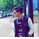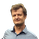80 views
80
The figure shows two exchange rate movements. I would not say fractal structure because the second structure is smaller than the first one. or just the similarity that characterizes the fractals. Why can you turn? Because the first structure over the wave axis was 15% closed, until the second structure was 30%. So the exchange rate rise in the second case is double the first. It is clear from the diagram that wave waveforms (green line) were formed in both cases, but at first the ATR axis (purple line) was the axis of resistance, and the second the ATR axis (purple line) support. That is why we assume that the exchange rate has reached the end of its correction level and that it can start to grow steadily.what are there rectangles for and what is the strategy called ?